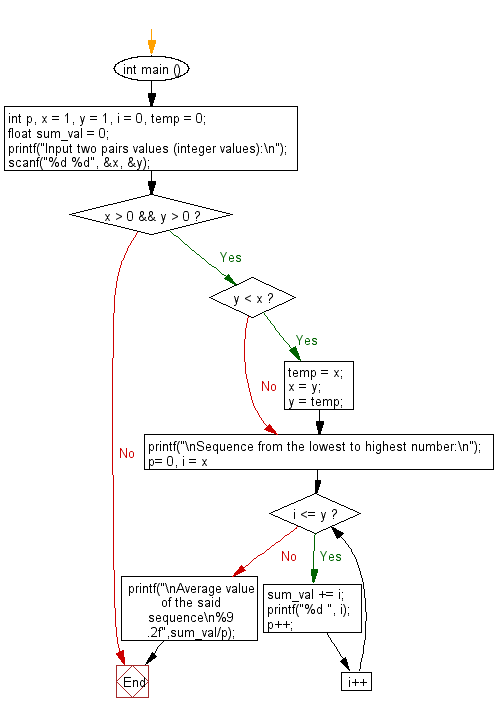﻿ C : Sequence from lowest to highest number and average value

# C Exercises: Sequence from the lowest to highest number and average value of the sequence

## C Basic Declarations and Expressions: Exercise-115 with Solution

Write a C program that accepts a pair of numbers from the user and prints the sequence from the lowest to the highest number. Also, print the average value of the sequence.

Sample Solution:

C Code:

``````#include <stdio.h>

int main () {
int p, x = 1, y = 1, i = 0, temp = 0;
float sum_val = 0;

// Prompt user for input
printf("Input two pairs values (integer values):\n");

// Read two integer values from user and store them in 'x' and 'y'
scanf("%d %d", &x, &y);

// Check if both 'x' and 'y' are positive
if (x > 0 && y > 0) {
// Swap 'x' and 'y' if 'y' is smaller than 'x'
if (y < x) {
temp = x;
x = y;
y = temp;
}
printf("\nSequence from the lowest to highest number:\n");

// Loop to print and accumulate values from 'x' to 'y'
for (p= 0, i = x; i <= y; i++) {
sum_val += i; // Accumulate the values for average calculation
printf("%d ", i);
p++;
}

// Print the average value of the sequence
printf("\nAverage value of the said sequence\n%9.2f", sum_val / p);
}

return 0; // End of program
}
``````

Sample Output:

```Input two pairs values (integer values):
14
25

Sequence from the lowest to highest number:
14 15 16 17 18 19 20 21 22 23 24 25
Average value of the said sequence
19.50
```

Sample Output:

```Input two pairs values (integer values):
35
13

Sequence from the lowest to highest number:
13 14 15 16 17 18 19 20 21 22 23 24 25 26 27 28 29 30 31 32 33 34 35
Average value of the said sequence
24.00
```

Flowchart:C programming Code Editor:

What is the difficulty level of this exercise?

Test your Programming skills with w3resource's quiz.

﻿